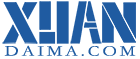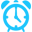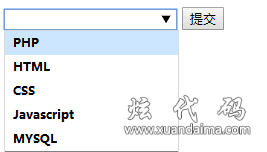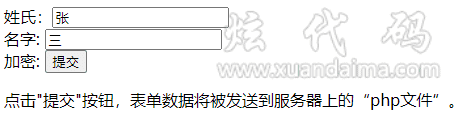# HTML5 新表单元素

HTML5 有以下新的表单元素:

• <datalist>

• <keygen>

• <output>注意:不是所有的浏览器都支持HTML5 新表单元素，但是你可以在使用它们，即使浏览器不支持表单属性，仍然可以显示为常规的表单元素。

## HTML5 <datalist> 元素

<datalist> 元素是指<input> 元素预定义选项列表。

<datalist> 元素用于提供一个带有"自动完成"特性的<input> 元素. 用户输入内容时会看到下拉选项。```<!DOCTYPE html>
<html>
<meta charset="utf-8">
<title>炫代码(www.xuandaima.com)</title>

<body>
<form action="/Template/Test/php/datalist.php">
<input list="lists" name="ming">
<datalist id="lists">
<option value="PHP">
<option value="HTML">
<option value="CSS">
<option value="JavaScript">
<option value="MYSQL">
</datalist>
<input type="submit">
</form>
<p><strong>注意:</strong> Internet Explorer 9（更早 IE 版本），Safari 不支持 datalist 标签。</p>

</body>
</html>```

## HTML5 <keygen> 元素

<keygen> 元素的作用是提供一种验证用户的可靠方法。

<keygen>标签规定用于表单的密钥对生成器字段。```<form action="/Template/Test/php/form_action.php">
姓氏：<input type="text" name="xing" value="张"><br>
名字: <input type="text" name="ming" value="三"><br>
加密: <keygen name = "random_key">
<input type="submit" />
</form>```

## HTML5 <output> 元素

<output> 元素表述计算的结果(有点像执行js脚本)。```<form onchange="ming.value=parseInt(a.value)+parseInt(b.value)">
<input type="number" id="a" value="50">
+<input type="number" id="b" value="50">
=<output name="ming" for="a加b结果"></output>
</form>```

HTML5 新表单元素

<datalist><input>标签定义选项列表。请与 input 元素配合使用该元素，来定义 input 可能的值。
<keygen><keygen> 标签规定用于表单的密钥对生成器字段。
<output><output> 标签定义不同类型的输出，比如脚本的输出。